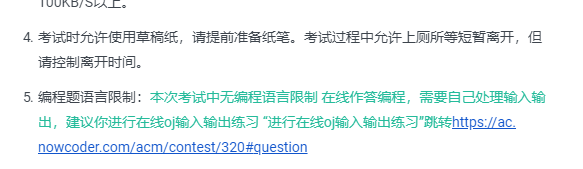# 数轴相邻点问题

## 题目描述

s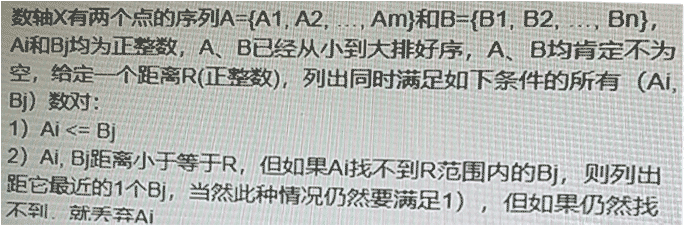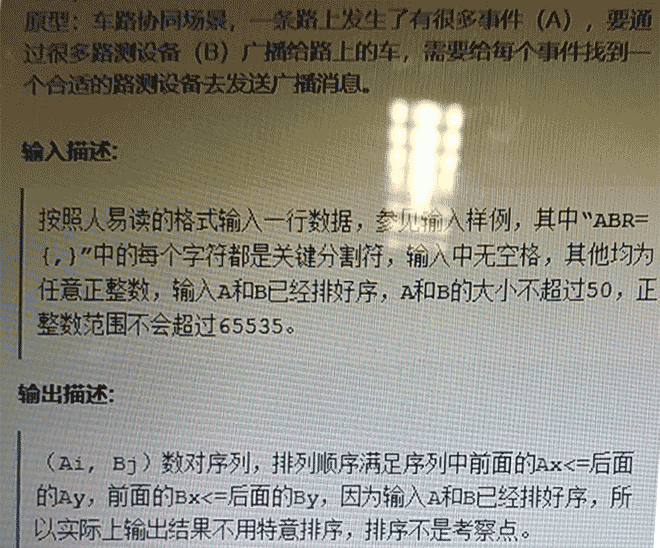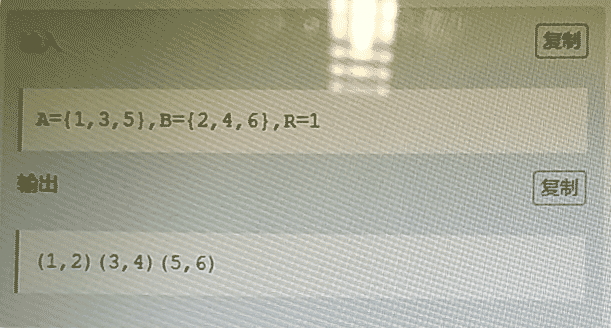## 解答

  1 2 3 4 5 6 7 8 9 10 11 12 13 14 15 16 17 18 19 20 21 22 23 24 25 26 27 28 29 30 31 32 33 34 35 36 37 38 39 40 41 42 43 44  s=input() A=[] B=[] R=0 area="" s=s.replace("{","").replace("}","").replace("=",",") for x in s.split(","): if x in "ABR": area=x elif x in "={}": pass else: if area=="A": A.append(int(x)) elif area=="B": B.append(int(x)) elif area=="R": R=int(x) else: raise ValueError i=0 j=0 na=len(A) nb=len(B) sj=0 while iB[sj] : sj+=1 if sj>=nb: break j=sj while j

# 字符串倒序排列问题

## 题目描述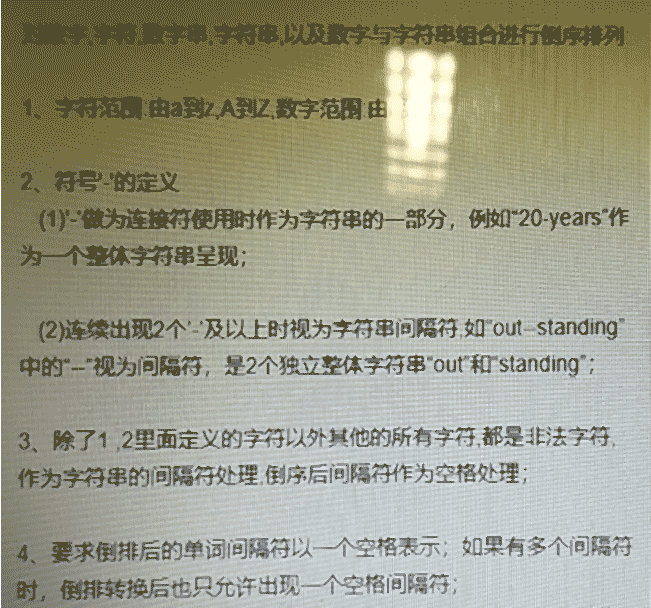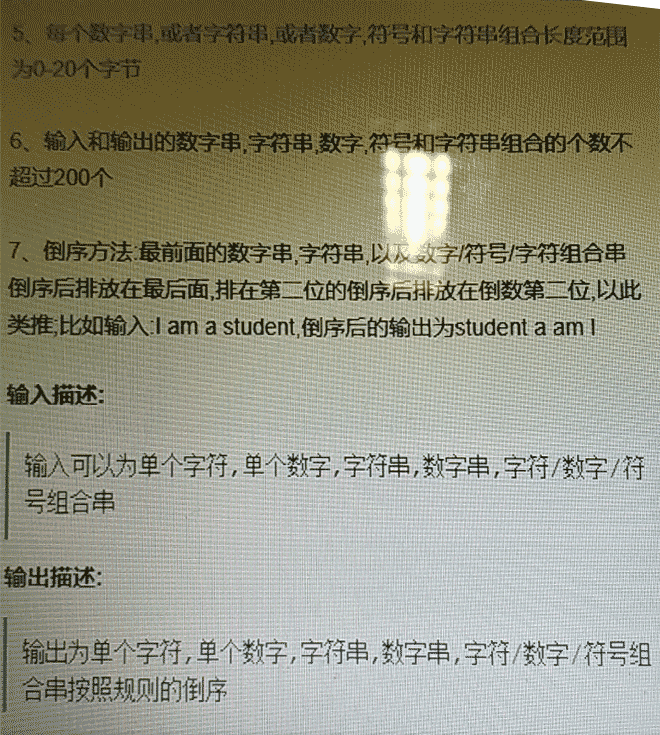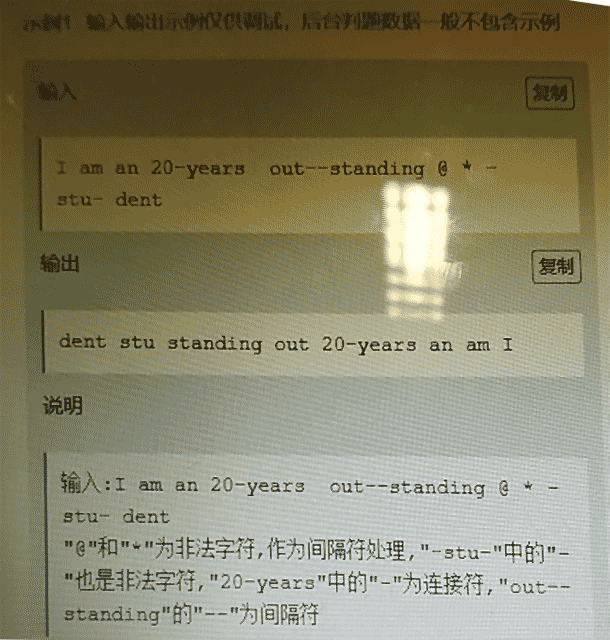## 解答

  1 2 3 4 5 6 7 8 9 10 11 12 13 14 15 16 17 18 19  import string CHR=string.digits+string.ascii_letters s=input() l=[] n=len(s) i=0 while i

# 航班线路问题

## 题目描述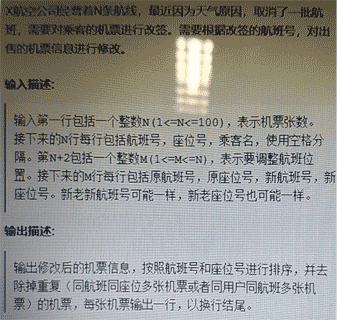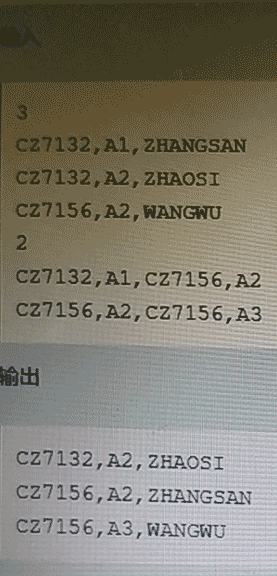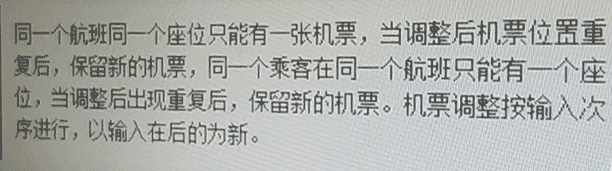## 解答

  1 2 3 4 5 6 7 8 9 10 11 12 13 14 15 16 17 18 19 20 21 22 23 24 25 26  N=int(input()) old={} for _ in range(N): fid,sid,name=input().split(',') old[(fid,sid)]=name new={} M=int(input()) names={} for _ in range(M): ofid,osid,nfid,nsid=input().split(',') ofs=(ofid,osid) nfs=(nfid,nsid) name=old.pop(ofs,) if names.get(name,("",""))==nfid: new.pop(names[name],) new[(nfid,nsid)]=name names[name]=(nfid,nsid) for fs,name in old.items(): if not (fs in new or names.get(name,("",""))==nfid) : new[fs]=old[fs] l=sorted(list(new.items()),key=lambda x: x) for fs,name in l: print("%s,%s,%s"%(fs,fs,name))